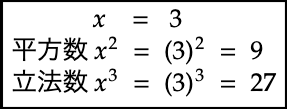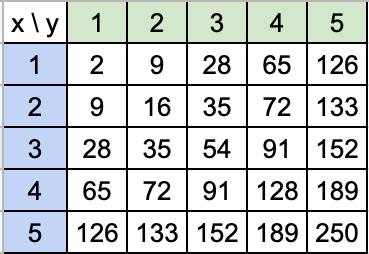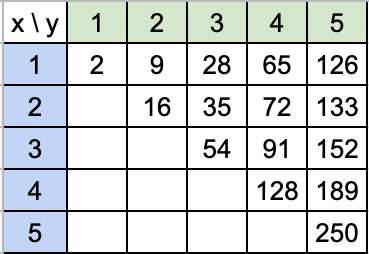# I checked the number of taxis with Ruby

The second in a series of verifications of mysterious numbers with Ruby! (How many more times will it continue?)

Click here for the previous 1st ↓ I checked with Ruby whether there is a Kaprekar number in an integer in a certain range

This time it is ** taxi number **. Like last time, I received the material from Youtube.

"University Mathematics / Physics" Learned at Preparatory School Familiar with Ramanujan's genius episode [Taxi number]

# What is the number of taxis?

The nth taxi number (denoted as taxi, taxicab number, Ta (n) or Taxicab (n)) is the smallest positive represented in n ways as the sum of ** two cubes **. Is defined as an integer of. The name "taxi number" comes from an episode in which Srinivasa Ramanujan pointed out that it was Ta (2) for the taxi number 1729 that Hardy took.

Source: Wikipedia

A ** cube number ** is a number that is the cube of a certain number **. Since the square number is squared, it's an evolutionary system.And the number of taxis seems to be expressed in several ways by the sum of the legislative numbers (addition).

# I looked it up

Contents: The smallest positive integer represented by n ways with `x ^ 3 + y ^ 3` (including the output of the combination of` x, y`) Condition: `(1 ≤ x ≤ y ≤ 500)`

#### `taxi.rb`

``````
def safe_invert(orig_hash)
orig_hash.each_key.group_by { |key| orig_hash[key] }.sort.to_h
end

hash = {}

(1..500).each do |y|
(1..500).each do |x|
hash.store([x, y], x ** 3 + y ** 3)
if x == y
break
end
end
end

taxi = []
n = 1

safe_invert(hash).each do |k, v|
if v.size == n
taxi.push("Ta(#{n}) => #{k}: #{v}")
n += 1
end
end

puts taxi
``````
``````Ta(1) => 2: [[1, 1]]
Ta(2) => 1729: [[9, 10], [1, 12]]
Ta(3) => 87539319: [[255, 414], [228, 423], [167, 436]]
``````

The minimum number that can be expressed in three ways as the sum of the cube numbers is already 87.53 million, which is quite a large number.

# Code description

I will explain step by step Let's try reducing the range for simplicity

Condition: `(1 ≤ x ≤ y ≤ 5)`

#### `taxu.rb`

``````
hash = {}

(1..5).each do |y|
(1..5).each do |x|
hash.store([x, y], x ** 3 + y ** 3)
end
end

p hash
``````
``````{[1, 1]=>2, [2, 1]=>9, [3, 1]=>28, [4, 1]=>65, [5, 1]=>126,
[1, 2]=>9, [2, 2]=>16, [3, 2]=>35, [4, 2]=>72, [5, 2]=>133,
[1, 3]=>28, [2, 3]=>35, [3, 3]=>54, [4, 3]=>91, [5, 3]=>152,
[1, 4]=>65, [2, 4]=>72, [3, 4]=>91, [4, 4]=>128, [5, 4]=>189,
[1, 5]=>126, [2, 5]=>133, [3, 5]=>152, [4, 5]=>189, [5, 5]=>250}
``````

First, give `x` and` y` an integer from 1 to 5.

Let's brute force the answer (sum), which is the sum of the cubed numbers, and add it to `hash`. The `store` method of the Hash class is a method that adds an element with key as the first argument and value as the second argument.

There are 5 x 5 = 25 different lengths for `hash`.As you can see from the table, the answer you get by swapping x and y is the same. The answer is `133` both when`(x, y) = (2, 5)`and when`(x, y) = (5, 2)`. This is not counted as two ways. There is one way. So it is this range that needs to be found in the tableSo, if `x` and` y` are the same, add an element to `hash` and` break` to break the loop.

#### `taxu.rb`

``````
hash = {}

(1..5).each do |y|
(1..5).each do |x|
hash.store([x, y], x ** 3 + y ** 3)
if x == y
break
end
end
end

p hash
``````
``````{[1, 1]=>2,
[1, 2]=>9, [2, 2]=>16,
[1, 3]=>28, [2, 3]=>35, [3, 3]=>54,
[1, 4]=>65, [2, 4]=>72, [3, 4]=>91, [4, 4]=>128,
[1, 5]=>126, [2, 5]=>133, [3, 5]=>152, [4, 5]=>189, [5, 5]=>250}
``````

Then the second half Especially the inside of the method is complicated.

In the hash, the key contained an array of `[x, y]`, and the value contained an element of `sum of cubes`. Passing through the `safe_invert method` creates a hash with the key and value reversed, taking into account duplication.

#### `taxi.rb`

``````
def safe_invert(orig_hash)
orig_hash.each_key.group_by { |key| orig_hash[key] }.sort.to_h
end

p safe_invert(hash)
``````
``````{2=>[[1, 1]], 9=>[[1, 2]], 16=>[[2, 2]], 28=>[[1, 3]], 35=>[[2, 3]],
54=>[[3, 3]], 65=>[[1, 4]], 72=>[[2, 4]], 91=>[[3, 4]], 126=>[[1, 5]],
128=>[[4, 4]], 133=>[[2, 5]], 152=>[[3, 5]], 189=>[[4, 5]], 250=>[[5, 5]]}
``````

`each_key.group_by { |key| orig_hash[key] }`With each key to value, ʻOrig_hash [key] `(that is, value) is used as the key to create a hash. Inversion of key value.

It's a difficult idea, but I referred to this Hash # invert (Ruby 2.7.0 Reference Manual)

And since it is sorted in ascending order of key with `sort` and at the same time it is converted to an array, Revert to hash with `.to_h`.

I think that the merit of this processing does not come to the fore when `x, y` is 1 to 5. If you extend the range from 1 to 12 so that the `Ta (2)` ** 1729 ** appears, the output result will look like this ...

``````{ ......, 1729=>[[9, 10], [1, 12]], ......}
``````

You can see that the length of the array of values is now two, and there are two ways to express it as the sum of the 1729 cubes.

#### `taxi.rb`

``````
taxi = []
n = 1

safe_invert(hash).each do |k, v|
if v.size == n
taxi.push("Ta(#{n}) => #{k}: #{v}")
n += 1
end
end

puts taxi
``````

With ʻeach`for the last hash Add the first matching array length of value from 1 to the empty array`taxi` Output the result and finish.

# Digression

I found out by looking at Wikipedia `Ta (4)` will not appear unless the range is expanded from ** 1 to 19000 **.

I tried running it with `(1..19000)`, Even after waiting for a few minutes, the process did not end and I gave up.

After all Ruby may not be suitable for exhaustive verification: sweat:

If you have a better way to write it, let us know in the comments!

** (Added on 2020/07/05) ** You suggested an improved version in the comments!